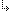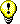- 2.2.5.1. -2. TMT Pascal Language Description2.2. Pascal Language Structure2.2.5. Constants2.2.5.1. Integer and Real Number Constants

## 2.2.5.1. Integer and Real Number Constants

Integer constants are values that can be represented in either decimal (base 10) or hexadecimal (base 16). A decimal number is a string of digits (0-9) that may be preceded with a plus or minus sign. A hexadecimal number is preceded by a dollar sign (\$) followed by a string of digits and the characters A through F. The following are valid integer numbers:
`  100   -255   100500   \$FE   \$ABCD`
Real constants are numbers that contain an integer portion, a fractional portion, and an exponent. Use real constants when the fraction of a number is necessary. The syntax for real constants is as follows:
`  [+|-]  digits [.digits] [E [+|-] digits]`
The letter E represents the exponent part of the real number. Exponents are powers of ten. Both integer and real constants may not contain space characters. The following are valid real constants:
`  1.0   -205.13   9019.31E100   40.71E-10`The current version of TMT Pascal compiler does not provide constants of Extended type.

- 2.2.5.1. -